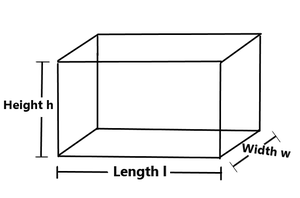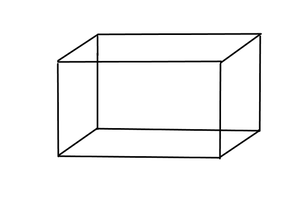Open in App
Not now

## Related Articles

• CBSE Class 9 Syllabus (All Subjects)
• CBSE Class 9 Maths Notes
• CBSE Class 9 Science Revision Notes
• CBSE Class 9 Social Science Revision Notes

# Volume of a Cuboid Formula

• Last Updated : 10 Jun, 2022

In geometry, a cuboid is a three-dimensional shape. A convex polyhedron surrounded by 6 rectangular faces with 8 vertices and 12 edges is called a cuboid. A cuboid with 6 square faces is called a cube. An example of a cuboid in real life is a rectangular box.

In mathematics, we can observe other shapes that are exactly like a cuboid. In geometry, a cuboid is a hexagon. Its face is a rectangle. “Cuboid” means “like a cube” in the sense that you can convert a cuboid into a cube by adjusting the length of the edges or the angle between the edges and faces. In the language of mathematics, a cuboid is a convex polyhedron whose polyhedral graph is the same as that of a cube.

### What is a Cuboid?

A cuboid is a 3D solid with 6 faces (rectangles), 8 vertices, and 12 edges. A cuboid has three dimensions: length, width, and height. A perfect cuboid is a cuboid with integer edges.

We know that a rectangle is a two-dimensional figure with four sides. Now imagine the shape formed when many congruent rectangles are placed on top of other rectangles. This shape is called a cuboid. Notice the following cuboid showing three dimensions: length, width, and height.### Shape of Cuboid

The shape of a cuboid is a closed 3D shape surrounded by a rectangular surface, that is, a flat area of ​​a rectangle. The shape of the cuboid is shown in the figure below.Cuboid

### Volume of Cuboid

The volume of a cuboid is the total space that the box occupies in three-dimensional space. A cuboid is a three-dimensional structure with six rectangular faces. These six faces of a cuboid exist as a pair of three parallel faces. Therefore, volume is a measure based on the dimensions of these faces: length, width, and height. It is measured in cubic units.

Volume of cuboid = length × width × height (cubic unit)

### How to calculate the volume of a cuboid?

The volume of a cuboid is the space it occupies inside the cuboid. A cuboid is a cube if all three dimensions of a cuboid are equal. The volume of a cuboid can be calculated using the cuboid volume formula. Steps to calculate the volume of a cuboid:

Step 1: Check that the dimensions of the given cuboid are in the same units. If not, convert the dimensions to the same units.

Step 2: Multiply the length, width, and height of the cuboid when the dimensions are in equal units.

Step 3: After you get the value, record the unit at the end.

### Sample Questions

Question 1: How to find the length of a cuboid?

The volume of a cuboid is equal to length × width × height.

Given the volume, width, and height,

We can write

v = l×b×h

l=v/bh

Question 2: Does the order of the cuboid matter for volume calculations?

It doesn’t matter whether you hold the cuboid vertically or horizontally. The volume of a cuboid is the same even if you change the order of length, width, and height.

Question 3: If you cut the length of one side by half, how will the volume of the cuboid change?

The volume of a cuboid is l = l/2, so if you cut the length of one side by half, it is halved.

Because volume = length × width × height

= (l/2) × b × h

= (lbh)/2

= volume/2.

Thus, the volume of a cuboid is halved as soon as its length is halved.

Question 4: If a cube has a volume of 3000 cm3, a width of 10 cm, and a height of 10 cm, what is the length?

The volume of a cuboid is Volume = length × width × height

The dimensions of a cuboid are as follows:

Volume=3000 cm3

Width=10 cm

Height=5 cm

The length of the cuboid =x cm.

So the volume of a cuboid is,

Volume=h×10×5=3000

h=3000/50

=60 cm.

The length of a cuboid is 60 cm.

Question 5: Cuboidal fish tanks have dimensions of 30 inches, 20 inches, and 15 inches. Can you determine the volume of an aquarium with fish?

Given that,

The Length of the aquarium = 30 inches

The Width of the aquarium = 20 inches

The Height of the aquarium = 15 inches

Since the volume of the aquarium is expressed as:

Volume = Length × Width × Height

= 30 × 20 × 15 inches3 = 9000 inches3

Thus, the tank has a volume of 9000 cubic inches.

My Personal Notes arrow_drop_up